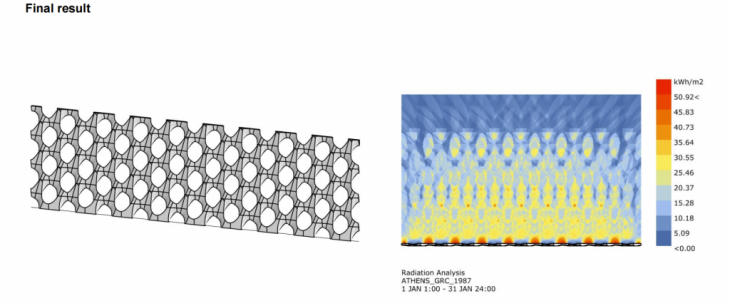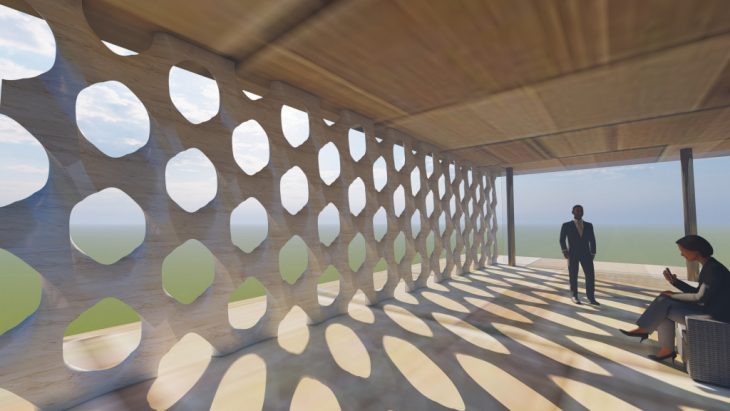Concept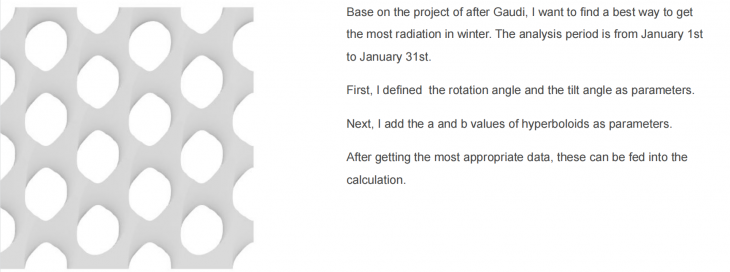First test

Parameters are rotating angle and tilting angle.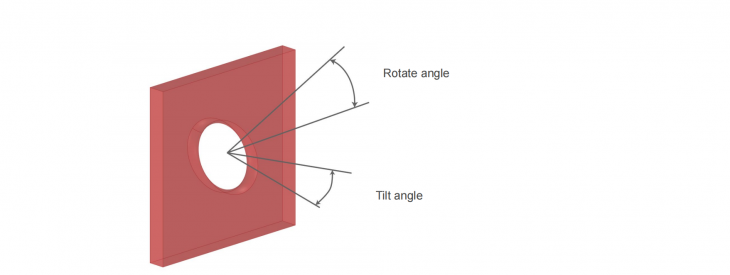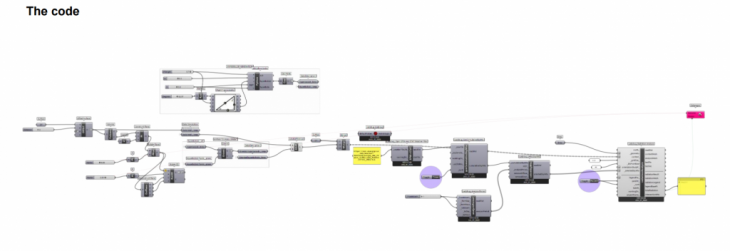Calculating GIF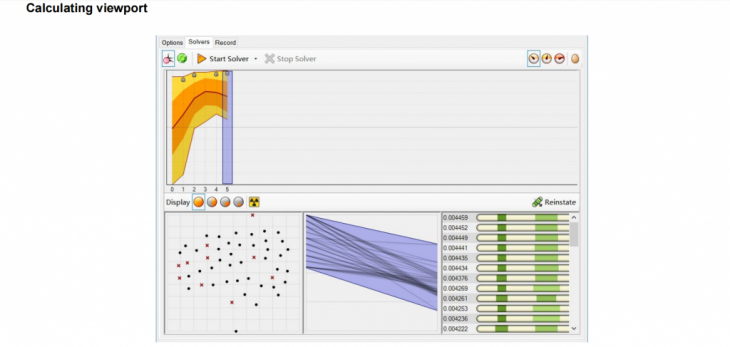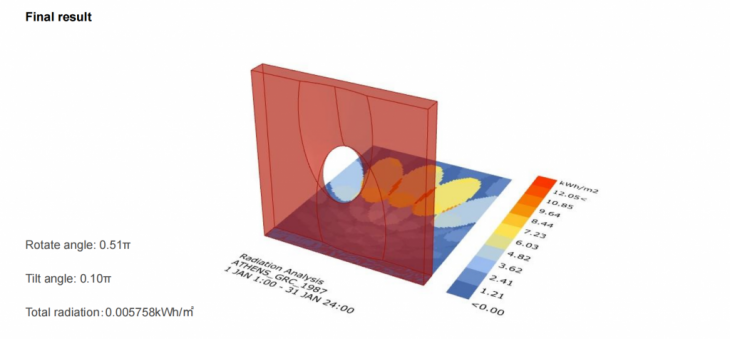Second test

Parameters are rotating angle, tilting angle, a and b.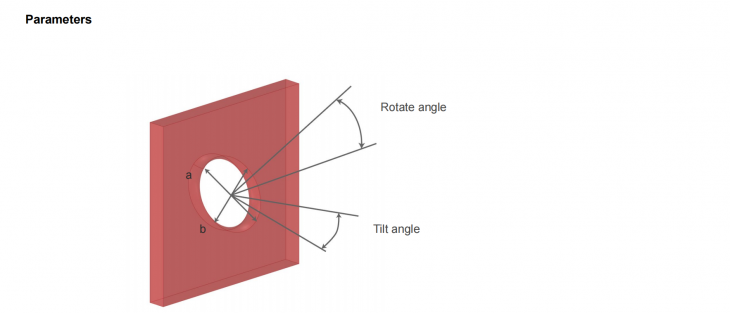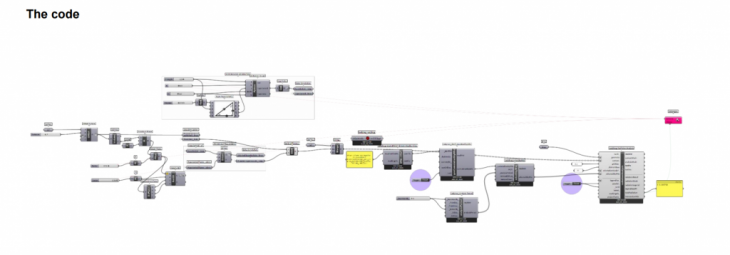Calculating GIF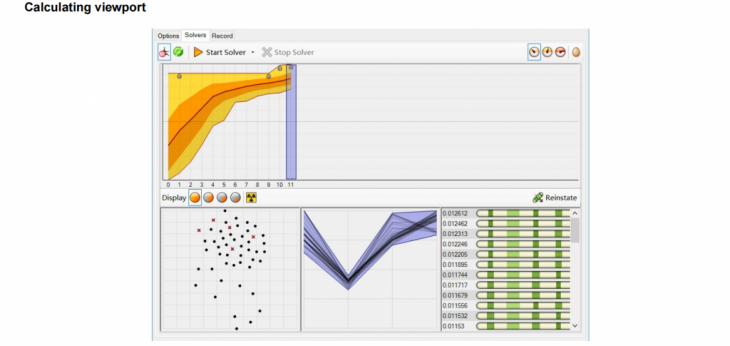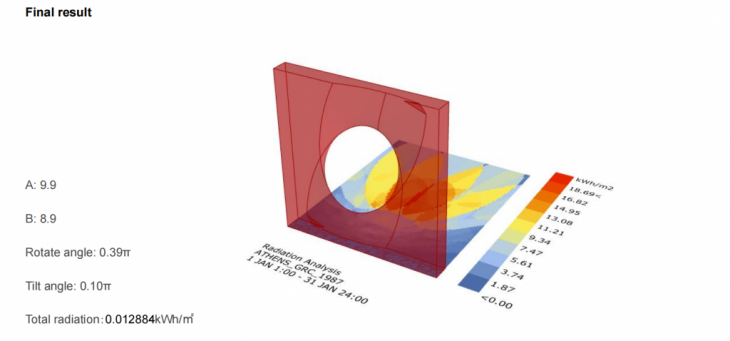The wall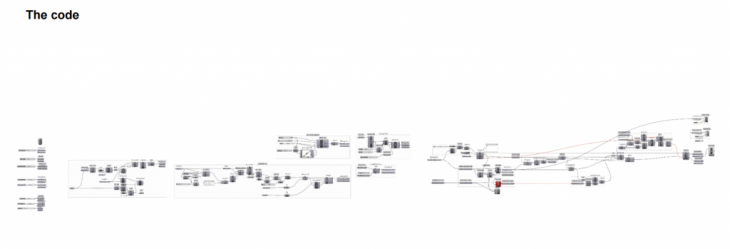Calculating GIF